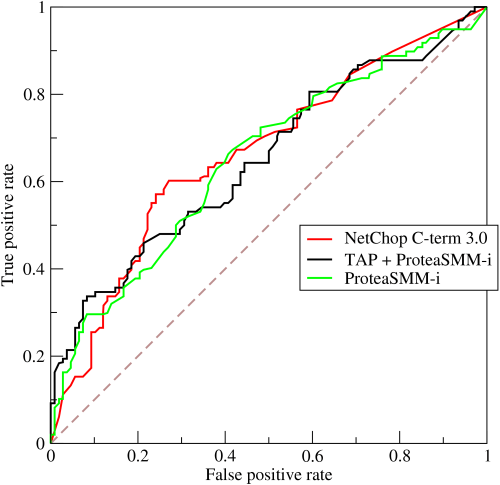理解ROC和AUC

ROC和AUC平时用的比较多，但是其真正的原理和计算过程却了解的不多，因此做个整理

• True Positive Rate(TPR): 60/(60+40)=0.6
• False Positive Rate(FPR): 140/(9760+140)=0.0141
• accuracy: (9760+60)/10000=0.982
• precision: 60/(60+140)=0.3
• recall: 60/100=0.6• sensitivity = recall = True Positive Rate
• specificity = 1- False Positive Rate• 先有一组真实分类，比如0,1,0,1；然后一组模型预测的打分（概率），比如：0.2,0.3,0.5,0.8
• 依据上述打分依次计算每个样本输出概率下的FPR和TPR
• 首先截断点为0.2，当概率大于等于0.2时，则预测为正例，因此4个样本均为正例（1），这时的FPR=1，TPR=1
• 接着截断点为0.3，因此当概率大于等于0.3时预测为正例，因此样本1预测为反例，样本2-4为正例，这时的FPR=0.5，TPR=1
• 以上述方法计算截断点0.5和0.8，当为0.5时，FPR=0.5，TPR=0.5；当为0.8时，FPR=0，TPR=0.5

library(ROCR)
y <- c(0,1,0,1)
p <- c(0.2,0.3,0.5,0.8)
pred <- prediction(p, y)
perf <- performance(pred, "tpr", "fpr")
perf
> perf
An object of class "performance"
Slot "x.name":
 "False positive rate"

Slot "y.name":
 "True positive rate"

Slot "alpha.name":
 "Cutoff"

Slot "x.values":
[]
 0.0 0.0 0.5 0.5 1.0

Slot "y.values":
[]
 0.0 0.5 0.5 1.0 1.0

Slot "alpha.values":
[]
 Inf 0.8 0.5 0.3 0.2

x.values对应FPR，y.values对应TPR, alpha.values对应预测打分cutoff，结果跟上面完全一致，然后简单做个ROC图，个人喜欢pROC包的图。。。

library(pROC)
modelroc <- roc(y,p)
plot(modelroc, print.auc=TRUE, auc.polygon=TRUE)• 从早期的计算ROC曲线下的面积
• 现在的可以通过计算正例score大于反例score的概率；比如上面例子中正例有2个(M)，反例有2个(N)，那么总共有2*2=4对（[0.3,0.2],[0.8,0.2],[0.3,0.5],[0.8,0.5]）正反样本对，同时通过其模型预测的score可看出正例score大于反例的有3对（[0.3,0.2],[0.8,0.2],[0.8,0.5]），因此AUC则为3/4=0.75
• 当样本比较多时，上述算法的复杂度过高O(N*M)，有人提出一个简单的算法：对score从到小排序，最大的score排序索引为n，最小的则为1；然后将正例的索引求和，减去正例-正例这种组合的个数M*(M+1)/2；最后除以M*N。以上述为例：正例的排序索引为2和4，M和N都为2，因此AUC=(2+4-2*(2+1)/2)/(2*2)=0.75